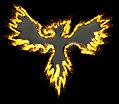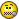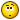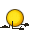###Author Topic: Enter the Matrix, Converting any matrix to GLBasic mat  (Read 6941 times)

####Hemlos

• To boldy go where no pixel has gone before!
• Global Moderator
• Prof. Inline
••• Posts: 1635
• Particle Hawk##### Enter the Matrix, Converting any matrix to GLBasic mat
« on: 2009-Nov-30 »
How do i convert a standard(is there one?) rotation matrix m to the GLBasic mat?

The help file says :

| a0  a4  a8 a12 |
| a1  a5  a9 a13 |
| a2  a6 a10 a14 |
| a3  a7 a11 a15 |

But i dont understand it.

Is mat a1 or a4?
mat[columns][rows]?
mat[rows][columns]?
« Last Edit: 2009-Nov-30 by Hemlos »
Volume_of_Earth(km^3) = 4/3*3.14*POW(6371.392896,3)

####Hemlos

• To boldy go where no pixel has gone before!
• Global Moderator
• Prof. Inline
••• Posts: 1635
• Particle Hawk##### Re: Enter the Matrix
« Reply #1 on: 2009-Nov-30 »
Answering myself, lol...It depends on the input, it needs to be converted to the opengl standard matrix.
So i put together 2 functions to convert any matrix from any function to the opengl matrix.
This is needed because youll find matrices on the internet written in one fashion or the other, as a matrix can be different from one language to the next.

« Last Edit: 2009-Nov-30 by Hemlos »
Volume_of_Earth(km^3) = 4/3*3.14*POW(6371.392896,3)

####Kitty Hello

• code monkey
• Prof. Inline
••• Posts: 10723
• here on my island the sea says 'hello'##### Re: Enter the Matrix, Converting any matrix to GLBasic mat
« Reply #2 on: 2009-Nov-30 »
The GLBasic, and thus OpenGL matrix is a set of 3 vectors (4 floats long, last one is zero) that describe direction and scale of x,y, and z axis:
The final vector is the translation:
[Xx, Xy, Xz, 0,  Yx, Yy, Yz, 0,  Zx, Zy, Zz, 0,  Tx, Ty, Tz, 1]
Where the captical letters are the axis, and the lowercase ones are the component of that axis's direction. Sort of...

####Hemlos

• To boldy go where no pixel has gone before!
• Global Moderator
• Prof. Inline
••• Posts: 1635
• Particle Hawk##### Re: Enter the Matrix, Converting any matrix to GLBasic mat
« Reply #3 on: 2009-Nov-30 »
Hmmm im trying to convert 4 vectors into the matrix to rotate an object.

The object is oriented so that the object is facing +z, and it top is facing +y.

The 4 vectors are defined as follows and never vary from thier original lengths, they are always 1 length in distance from each other and can rotate around the origin in 6 degrees of freedom.

The 4 vectors are always locally oriented to each other as follows:

A. an origin position in 3d (x,y,z)

B. a vector oriented to the right of origin, is locally -x direction

C. a vector oriented in front of the origin, is locally +z direction

D. a vector oriented directly above the origin, is locally +y direction

Again,  B C and D vectors are 1 unit length away from the origin.

Here is the local orientation as a diagram, how these positions are related:

// orientation                    vector desciption
// (C=0,1,0)                     up - locally +y in respect to origin
//        |  /(D=0,0,1)          look - locally + z in respect to origin
//        | /
//        |/___(B=-1,0,0)      right -  locally -x in respect to origin
//   (A=0,0,0)                    origin - local x y z

I can rotate all 3(BCD) around the origin in 6 degrees of freedom with quaternions...no problem...

I have tried the sequence of vector inputs into a matrix array, but they always seem to be attached to 0,0,0 no matter what.

Here is the code i have, converted with the function set below this:

Code: GLBasic [Select]
DIM m
m=cam1.rx
m=cam1.ry
m=cam1.rz
m=0

m=cam1.ux
m=cam1.uy
m=cam1.uz
m=0

m= cam1.lx
m= cam1.ly
m= cam1.lz
m=0

m=0
m=0
m=0
m=1

DIM mat
mXY_Matrix()
X_MOVEMENT x,y,z
X_PUSHMATRIX
X_MULTMATRIX mat[]

X_DRAWOBJ Object,0
X_POPMATRIX

This object is always attached in the middle of the object to point xyz (0,0,0)...the pushmatrix isnt moving the object....and the object is stretched out meeting at the look vector....and the object seems flat. I also tried using mYX_Matrix(), and it is messed up looking also, scaling the object up to huge proportions.

Can you help make a function to convert these vectors into the X_MULT, so it moves and rotates an object?

Here are the converters:
Code: GLBasic [Select]
//Where m = m[column][row] = m[x][y]
FUNCTION Opengl_XY_Matrix:
//opengl matrix

DIM mat

mat=  m
mat=  m
mat=  m
mat=  m

mat=  m
mat=  m
mat=  m
mat=  m

mat=  m
mat=  m
mat= m
mat= m

mat= m
mat= m
mat= m
mat= m

RETURN 1

ENDFUNCTION

Code: GLBasic [Select]
//Where m = m[row][column] = m[y][x]
FUNCTION Opengl_YX_Matrix:
//opengl matrix

DIM mat

mat=  m
mat=  m
mat=  m
mat=  m

mat=  m
mat=  m
mat=  m
mat=  m

mat=  m
mat=  m
mat= m
mat= m

mat= m
mat= m
mat= m
mat= m

RETURN 1

ENDFUNCTION
« Last Edit: 2009-Nov-30 by Hemlos »
Volume_of_Earth(km^3) = 4/3*3.14*POW(6371.392896,3)

####Kitty Hello

• code monkey
• Prof. Inline
••• Posts: 10723
• here on my island the sea says 'hello'##### Re: Enter the Matrix, Converting any matrix to GLBasic mat
« Reply #4 on: 2009-Dec-01 »
Code: GLBasic [Select]
//! Rotations: Roll, Yaw, Pitch and Offset by: x,y,z
//? Verdrehung: Roll-, Gier-, Nickwinkel und Verschiebung um: x,y,z
// \param rx - roll angle                       |Rollwinkel
// \param ry - yaw angle                        |Gierwinkel
// \param rz - pitch angle                      |Nickwinkel
// \param x  - offset x                         |Verschiebung in x
// \param y  - offset y                         |Verschiebung in y
// \param z  - offset z                         |Verschiebung in z
// \param M[] - [out] the transformation matrix |[Ausgabe]Die Transformationsmatrix
FUNCTION NewtonBuildMatrix: rx, ry, rz, x, y, z, M[]
LOCAL sa,sb,sc, ca,cb,cc
LOCAL sacb, cacb
DIM M
ca       = COS(-rx);
sa       = SIN(-rx);
sb       = COS(ry);
cb       = SIN(ry);
cc       = COS(-rz);
sc       = SIN(-rz);

sacb=sa*cb
cacb=ca*cb

M  =   sb * cc;
M  =  -sb * sc;
M  =  -cb;

M  = -sacb * cc + ca * sc;
M  =  sacb * sc + ca * cc;
M  =  -sa * sb;

M  =  cacb * cc + sa * sc;
M  = -cacb * sc + sa * cc;
M =   ca * sb;

M= x
M= y
M= z
M= 1;
ENDFUNCTION

####Hemlos

• To boldy go where no pixel has gone before!
• Global Moderator
• Prof. Inline
••• Posts: 1635
• Particle Hawk##### Re: Enter the Matrix, Converting any matrix to GLBasic mat
« Reply #5 on: 2009-Dec-01 »
This still wont represent a quaternion rotation, it is euler rotations.

Questions:

1. The camera uses glRotatef() to rotate the camera??
2. Objects use glRotatef(), to rotate an object?  X_ROTATION is glRotatef() ??

Is there a way to rotate an object, the same way we do it with a camera,(origin, pointto, up)Please say yes« Last Edit: 2009-Dec-02 by Hemlos »
Volume_of_Earth(km^3) = 4/3*3.14*POW(6371.392896,3)

####Kitty Hello

• code monkey
• Prof. Inline
••• Posts: 10723
• here on my island the sea says 'hello'##### Re: Enter the Matrix, Converting any matrix to GLBasic mat
« Reply #6 on: 2009-Dec-15 »
the camera just transforms the projection matrix. I'm using something like gluLookAt() for that.
The X_ROTATION is glRotate (basically). Internally I provide all that myself and call glMultMatrix, later, because that's faster.

####Hemlos

• To boldy go where no pixel has gone before!
• Global Moderator
• Prof. Inline
••• Posts: 1635
• Particle Hawk##### Re: Enter the Matrix, Converting any matrix to GLBasic mat
« Reply #7 on: 2009-Dec-15 »
Hmm, X_ROTATION gimbal lock problem is really bad when 1 of the 3 rotations are near 90 degrees.

I got this info from opengl...i dont understand it too well..
and gluLookAt is equivalent to glMultMatrixf(M); glTranslated (-eyex, -eyey, -eyez);

Is there a way to rotate objects like this too?

http://pyopengl.sourceforge.net/documentation/manual/gluLookAt.3G.html

Quote
Let

F=
centerX Ã¢Ë†â€™ eyeX
centerY Ã¢Ë†â€™ eyeY
centerZ Ã¢Ë†â€™ eyeZ

Let UP be the vector (upXupYupZ).

Then normalize as follows:

f=F
||F||

UP'=UP
||UP||

Finally, let s=fÃƒâ€”UP', and u=sÃƒâ€”f.

M is then constructed as follows:

M=
(s s s 0
u u u 0
-f -f -f 0
0 0 0 1
)

and gluLookAt is equivalent to glMultMatrixf(M); glTranslated (-eyex, -eyey, -eyez);
Volume_of_Earth(km^3) = 4/3*3.14*POW(6371.392896,3)